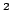Statistica Sinica 16(2006), 1273-1284

A NOTE ON THE LASSO AND RELATED PROCEDURES

IN MODEL SELECTION

Chenlei Leng, Yi Linand Grace WahbaNational University of Singapore andThe University of Wisconsin-Madison

Abstract: The Lasso, the Forward Stagewise regression and the Lars are closely related procedures recently proposed for linear regression problems. Each of them can produce sparse models and can be used both for estimation and variable selection. In practical implementations these algorithms are typically tuned to achieve optimal prediction accuracy. We show that, when the prediction accuracy is used as the criterion to choose the tuning parameter, in general these procedures are not consistent in terms of variable selection. That is, the sets of variables selected are not consistently the true set of important variables. In particular, we show that for any sample size, when there are superfluous variables in the linear regression model and the design matrix is orthogonal, the probability that these procedures correctly identify the true set of important variables is less than a constant (smaller than one) not depending on. This result is also shown to hold for two-dimensional problems with general correlated design matrices. The results indicate that in problems where the main goal is variable selection, prediction-accuracy-based criteria alone are not sufficient for this purpose. Adjustments will be discussed to make the Lasso and related procedures useful/consistent for variable selection.

Key words and phrases: Consistent model selection, Forward Stagewise regression, Lars, Lasso, variable selection.